# Getting Started with SHARC+ Assembly Programming, part 1: Introduction

2021-06-24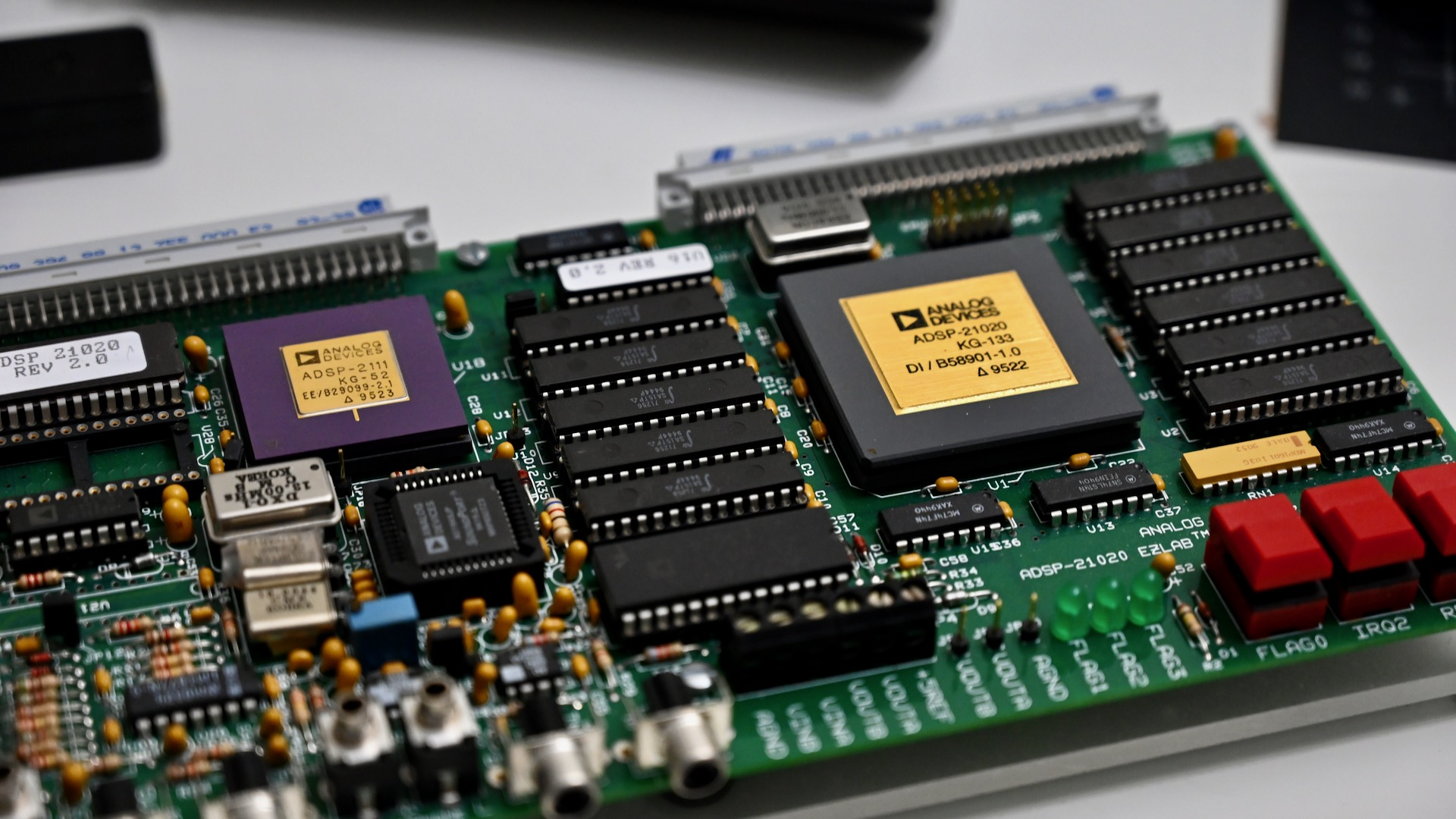# Prologue

During the past year, I found myself writing a lot of SHARC+ assembly code. For me, the SHARC is quite an interesting ISA. It's in some way very different from other general-purpose RISC processors. From a quick search, there is no SHARC+ assembly programming tutorial I can find online. As a result, I am writing one myself. This is more or less a quick guide instead of a complete document. For the latter, please read the official SHARC+ PRM (programming reference manual).

This guide assumes knowledge of the C programming language and common computer architecture. Familiarity with at least one kind of assembly language (x86, arm, MIPS, z80, etc.) would help a lot.

If you are intending to learn to program the SHARC+ DSP, hope this tutorial will help you. If you are just a computer architecture enthusiast like me, just wanting to learn about this architecture, hope you would find this tutorial interesting.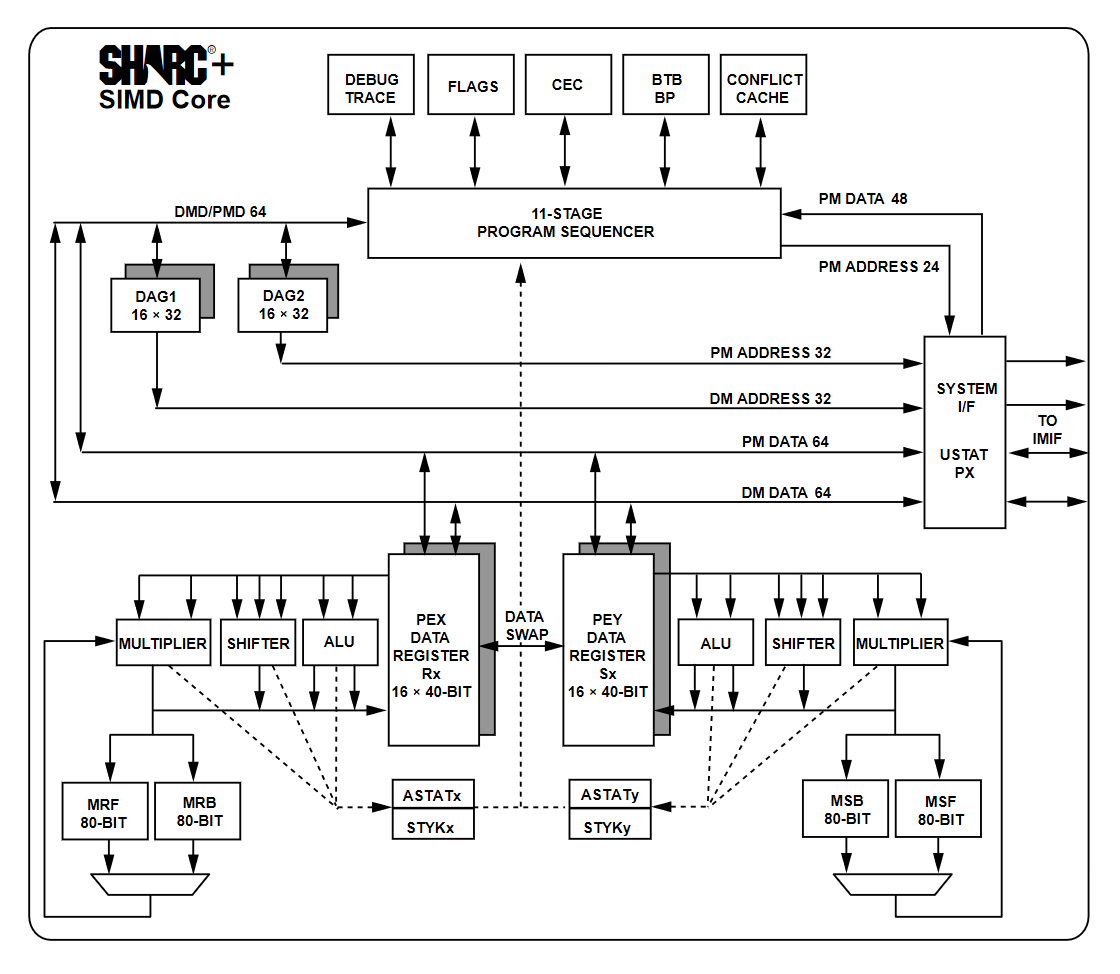(Figure: SHARC+ core diagram, source: SHARC+ PRM)

# Introduction

SHARC, which stands for Super Harvard Architecture Single-Chip Computer, is a series of DSPs designed and sold by Analog Devices Inc since 1991. By the time of writing (2021), there are 3 variants of the ISA:

• SHARC (correspond to SHARC-V core)
• TigerSHARC
• SHARC+ (correspond to SHARC-XI core)

This series of tutorials only apply to SHARC and SHARC+. All the processors in the 215xx series use the SHARC+ core. About the core name, SHARC-V simply means it has a 5-stage pipeline, and SHARC-XI simply means it has an 11-stage pipeline. SHARC+ is backward compatible with SHARC.

From a high level, SHARC and SHARC+ are RISC-like (load-store model) VLIW (vector processing) processors with SIMD (array processing) capability. We will talk about this more in the future.

One major characteristic about VLIW processors (including SHARC and other DSP ISAs) is that the microarchitecture design is intentionally exposed to the programmer so that the code could explicitly leverage hardware features provided by the processor. We shall see how SHARC's architecture would affect the way the code is being written.

In this series, I will only be focusing on the parts that are commonly used when implementing DSP algorithms. Generally, I will also only focus on floating-point algorithms.

For the tutorial, there will be many examples. A physical board is not required but could be helpful if you want to try out the code yourself. I will be using the SAM (SHARC Audio Module) board (also called SC589-MINI) and CCES (ADI's official IDE for SHARC+ DSPs) 2.10 throughout the tutorial. As of writing, the SAM board is available from Analog Devices for \$195 with bundled JTAG ICE and CCES license.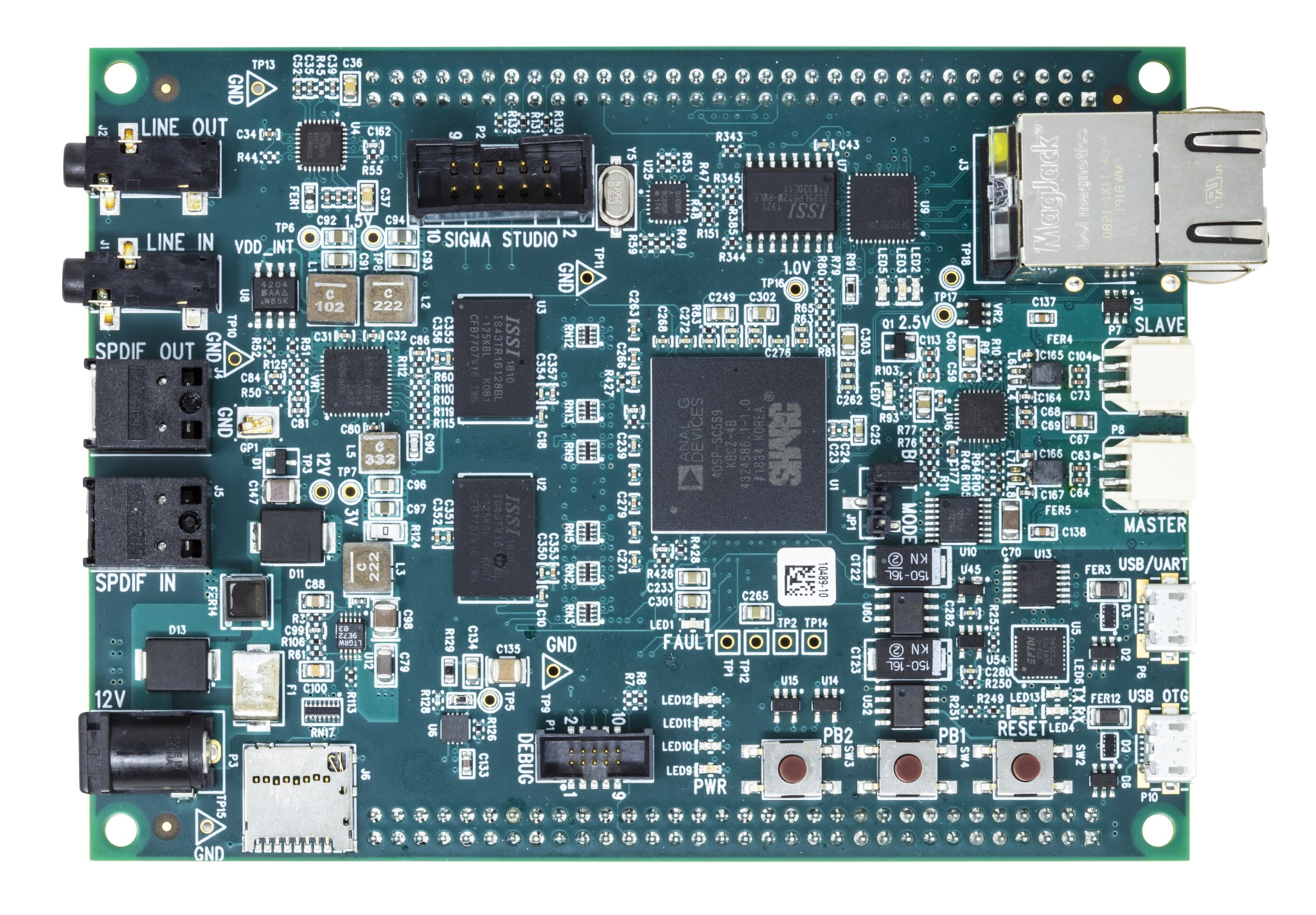(Figure: SAM board, source: https://wiki.analog.com/resources/tools-software/sharc-audio-module)

Let's get started!

# Registers

SHARC is a load-store machine. It means all the data needs to be loaded into registers before computation. There are a lot of registers, but here I am only going to outline ones that are commonly used in programming:

## Data Registers / Register File

The register file has 16 registers, named R0 to R15, and F0 to F15. The R version is used in fixed-point calculations, and the F version is used in floating-point calculations. Unlike on common RISC architectures, F registers are not separate registers, they are just alias for R registers and share the same physical register. For example, setting R5 to 0x12345678 will also set F5 to 0x12345678 (whatever that means in IEEE754). Note on SHARC these registers are actually 40bit wide, so you could get a larger range or higher precision when doing the calculation. There is also a hardware mode allowing you to limit the floating-point operation to only use 32bit.

## DAG Registers

Unlike common RISC machines, SHARC cannot address memory with data registers. This means you cannot simply load an address into some data register and access the memory by doing a load with that register. Instead, separate DAG (Data Address Generator) registers are provided for these purposes. There are 4 sets of DAG registers:

• I0-15, index registers
• M0-15, modify registers
• B0-15, base registers
• L0-15, length registers

I will talk about the usage in the memory access section.

## Other registers

There are many other registers in the processor. Few notable ones:

• MODE1/2, processor mode register
• LCNTR, the loop counter
• ASTAT, arithmetic status register (flags)

We will get to them once related features are being used. As always, you could find the full definition of all registers in the PRM.

# Calculations

Let's start with integer calculation. For example, add two numbers together. Normally in a typical RISC machine, you would expect some instruction like this: `ADD rdst, rsrc1, rsrc2`, which adds two registers and save the result into a 3rd register. On SHARC, it is a similar story, but the assembly syntax looks like this:

``````rdst = rsrc1 + rsrc2;
``````

So it looks more like C than assembly. But that's just the syntax, it is still assembly instead of C. On the SHARC/SHARC+, the following ALU calculations are supported:

• Rn = Rx + Ry (int and float)
• Rn = Rx - Ry (int and float)
• Rn = Rx + Ry, Rm = Rx - Ry (int and float, simultaneous add and subtract, must be the same pair of register)
• Rn = Rx + Ry + ci (int, add with carry)
• Rn = Rx - Ry + ci - 1 (int, subtract with carry)
• Rn = (Rx + Ry) / 2 (int and float)
• Rn = -Rx (int and float)
• Rn = abs Rx (int and float)
• Fn = abs (Fx + Fy) (float)
• Fn = abs (Fx - Fy) (float)
• Rn = pass Rx (int and float)
• Rn = min (Rx, Ry) (int and float)
• Rn = max (Rx, Ry) (int and float)
• Rn = clip Rx BY Ry (int and float, Rn = (|Rx| < |Ry|)?(Rx):((Rx >0 )?|Ry|:-|Ry|)
• Rn = Rx + ci (int)
• Rn = Rx + ci - 1 (int)
• Rn = Rx + 1 (int)
• Rn = Rx - 1 (int)
• Rn = Rx and Ry (int)
• Rn = Rx or Ry (int)
• Rn = Rx xor Ry (int)
• Rn = not Rx (int)
• Fn = rnd Fx (float, round to 32bit)
• Fn = scalb Fx by Ry (float, Fn = Fx * 2^Ry)
• Fn = recips Fx (float, creates an 8-bit accurate seed for 1/Fx)
• Fn = rsqrts Fx (float, creates an 4-bit accurate seed for 1/sqrt(Fx))
• Rn = mant Fx (float)
• Rn = logb Fx (float)
• Rn = fix Fx by Ry (Rn = (int)Fx * 2^Ry)
• Rn = fix Fx (Rn = (int)Fx)
• Fn = float Rx By Ry (Fn = (float)Rx * 2^Ry)
• Fn = float Rx (Fn = (float)Rx)
• comp (Rx, Ry) (int and float, compare and set flags)

That's a lot of operations. Some operations have both integer version and floating-point version, some only have 1 of them. The operation is distinguished by the register name (Rn for integer/fixed-point, and Fn for floating-point). But you may wonder, why there is no multiplication? On SHARC, the ALU doesn't support multiplication. Instead, there is a dedicated multiplier, supporting the following operations:

• Rn = Rx * Ry (int and float)
• Rn = MRF/MRB + Rx * Ry (int)
• Rn = MRF/MRB - Rx * Ry (int)
• Rn = sat MRF/MRB (int)
• Rn = rnd MRF/MRB (int)

MRF and MRB are special 80-bit integer result registers in the multiplier and could help with certain integer multiplications. Note there is no fused multiply-add (FMAC) for floating-point.

Well, what about bit shifts? There is also a shifter present, with a lot of bit operation instructions. I am not going to list them here but you could find them in the PRM.

Division is not natively supported, and needs to be implemented in software. We will talk more about division in the future.

# Function Call

I am sorry after so much stuff we still couldn't start writing our first code but believe we are getting close. After knowing how function calling works we could start writing the first simple function.

## Calling and Returning

There are 4 instructions for branches:

• CALL
• JUMP
• RTS
• RTI

Jump is just jumping to a location, call would also push the return address into the PC stack (PCSTK, a separate stack than the normal data stack) besides jumping. RTS is used to return from a subroutine, and RTI is used to return from interrupt. So basically the caller would use CALL to call a function, and the callee function would use RTS to return to the caller after finished.

Jump, call, and returns all have 2 delay slots. In case you are not familiar with delay slots, they are instructions that are always executed before branching (while the branch itself could be conditional). We will talk more about this later when optimizing the code.

## Calling Convention

The hardware handles the control transfer, but the software is responsible for data transferring between functions. The following is a brief calling convention for SHARC, you could always find the full convention by searching "registers" in CCES help.

• R4: 1st argument
• R8: 2nd argument
• R12: 3rd argument
• R0: return value
• R1: long return value
• R2: caller saved (scratch)
• All other data registers are callee saved
• I4/ I12/ I13: scratch
• I6: frame pointer
• I7: stack pointer
• All other index registers are callee saved
• M4/ M12: scratch
• M5/ M13: Always equal to 0
• M6/ M14: Always equal to 1
• M7/ M15: Always equal to -1
• All other modify registers are callee saved

# Example

## Running the C example

Finally, time for an example! For the 1st example, we are going to write a function that adds 2 numbers together in assembly. Because this is the first example, I am going to include more details about using the CCES and running on the board.

Use File->New->New CrossCore Project to create a new project. Leave all the configurations as default. We will only be using Core1 to run the code. (Core0 is the ARM core, and Core1 and Core2 are the 2 DSP cores). But, make sure you create projects for all 3 cores.

After the project has been created, create a new source file in Core1's src folder, called add_test.c. Copy & Paste the following code there:

```// add_test.c
#include <stdio.h>
#include <stdlib.h>
#include <math.h>
#include <assert.h>
#include <time.h>

#define BLOCKSIZE 256
#define MIN_SNR 100.f

// Generate an array of random integers of specified range
// Note: un-even coverage
void random_int(int *dst, int min, int max, int count) {
for (int i = 0; i < count; i++) {
dst[i] = rand() % (max - min) + min;
}
}

// Generate an array of random floating point numbers of specified range
// Note: generated random number only covers partial of possible numbers
void random_float(float *dst, float min, float max, int count) {
for (int i = 0; i < count; i++) {
dst[i] = (float)rand() / (float)RAND_MAX * (max - min) + min;
}
}

// Calculate SNR in dB of given signal against given reference signal
float snr_int(int *ref, int *signal, int count) {
float Ssqr = 0.f, Nsqr = 0.f;
for (int i = 0; i < count; i++) {
Ssqr += (float)ref[i] * (float)ref[i];
Nsqr += ((float)ref[i] - (float)signal[i]) *
((float)ref[i] - (float)signal[i]);
}
return (Nsqr == 0.f) ? (INFINITY) : (10.f * log10(Ssqr / Nsqr));
}

// Calculate SNR in dB of given signal against given reference signal
float snr_float(float *ref, float *signal, int count) {
// SNR = E[S^2] / E[N^2], where signal is the reference,
// and noise is the difference between signal and reference
float Ssqr = 0.f, Nsqr = 0.f;
for (int i = 0; i < count; i++) {
Ssqr += ref[i] * ref[i];
Nsqr += (ref[i] - signal[i]) * (ref[i] - signal[i]);
}
return (Nsqr == 0.f) ? (INFINITY) : (10.f * log10(Ssqr / Nsqr));
}

// Gold reference implementation of add function
int add_ref(int a, int b) {
return a + b;
}

// add function that will be tested
int add(int a, int b) {
return a + b;
}

// function to run the test
int *src_a;
int *src_b;
int *dst_ref;
int *dst;

src_a = malloc(sizeof(int) * BLOCKSIZE);
assert(src_a);
src_b = malloc(sizeof(int) * BLOCKSIZE);
assert(src_b);
dst_ref = malloc(sizeof(int) * BLOCKSIZE);
assert(dst_ref);
dst = malloc(sizeof(int) * BLOCKSIZE);
assert(dst);

random_int(src_a, -100, 100, BLOCKSIZE);
random_int(src_b, -100, 100, BLOCKSIZE);

clock_t cycles_ref = clock();
for (int i = 0; i < BLOCKSIZE; i++) {
}
cycles_ref = clock() - cycles_ref;

clock_t cycles = clock();
for (int i = 0; i < BLOCKSIZE; i++) {
}
cycles = clock() - cycles;

float snr = snr_int(dst_ref, dst, BLOCKSIZE);
printf("Finished in %d cycles (Ref %d cycles), SNR = %.f dB\n",
cycles, cycles_ref, snr);
if (snr < MIN_SNR) {
printf("Test failed! First 4 numbers in the result:\n");
for (int i = 0; i < 4; i++) {
printf("Result: %d, Ref: %d\n", dst[i], dst_ref[i]);
}
}

free(src_a);
free(src_b);
free(dst_ref);
free(dst);
}
```

That's a lot of code to run for just addition. Well, this includes a fairly simple but useful testing harness for the function. As you could see there are 2 Add functions, one called add, another called add_ref. The idea is that we would have a C version gold reference code. Our assembly version of the code should match the output of the C version. It also benchmarks the performance of the assembly code against the C version, which would be useful later when we get to the optimization part.

When comparing the result, note it is calculating the SNR instead of comparing the equality of numbers. This is because, in optimizations, we would often modify the order of calculation, and that would cause the result to be slightly different. So we only calculate the SNR and see if the results are close enough, and this is what we care about in signal processing anyway. Here, the signal is defined to be the gold reference output, and the noise is defined to be the difference between the gold reference output and function under test output.

Later it would be a good idea to move utility codes (`random_int`, `random_float`, `snr_int`, and `snr_float`) into a separate file.

Add the function call to add_test in main function, save and build.

When it is ready to run, select the Core0 project in the Project Explorer, click on the debug button to create a new debugging session: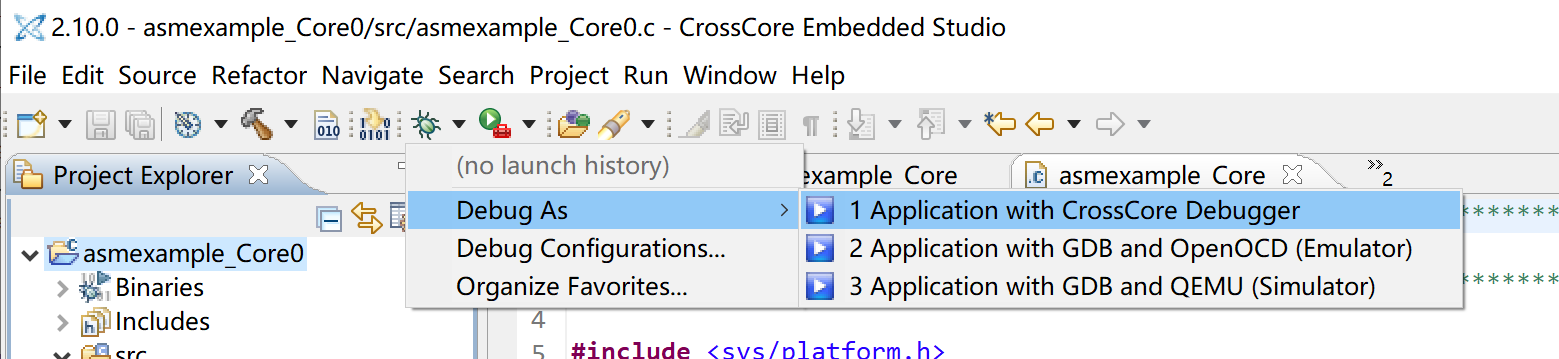In the Debug Configurations window, make sure the program is automatically selected for all 3 cores. If not, delete the debug session on the left, make sure the Core0 project is selected, and retry.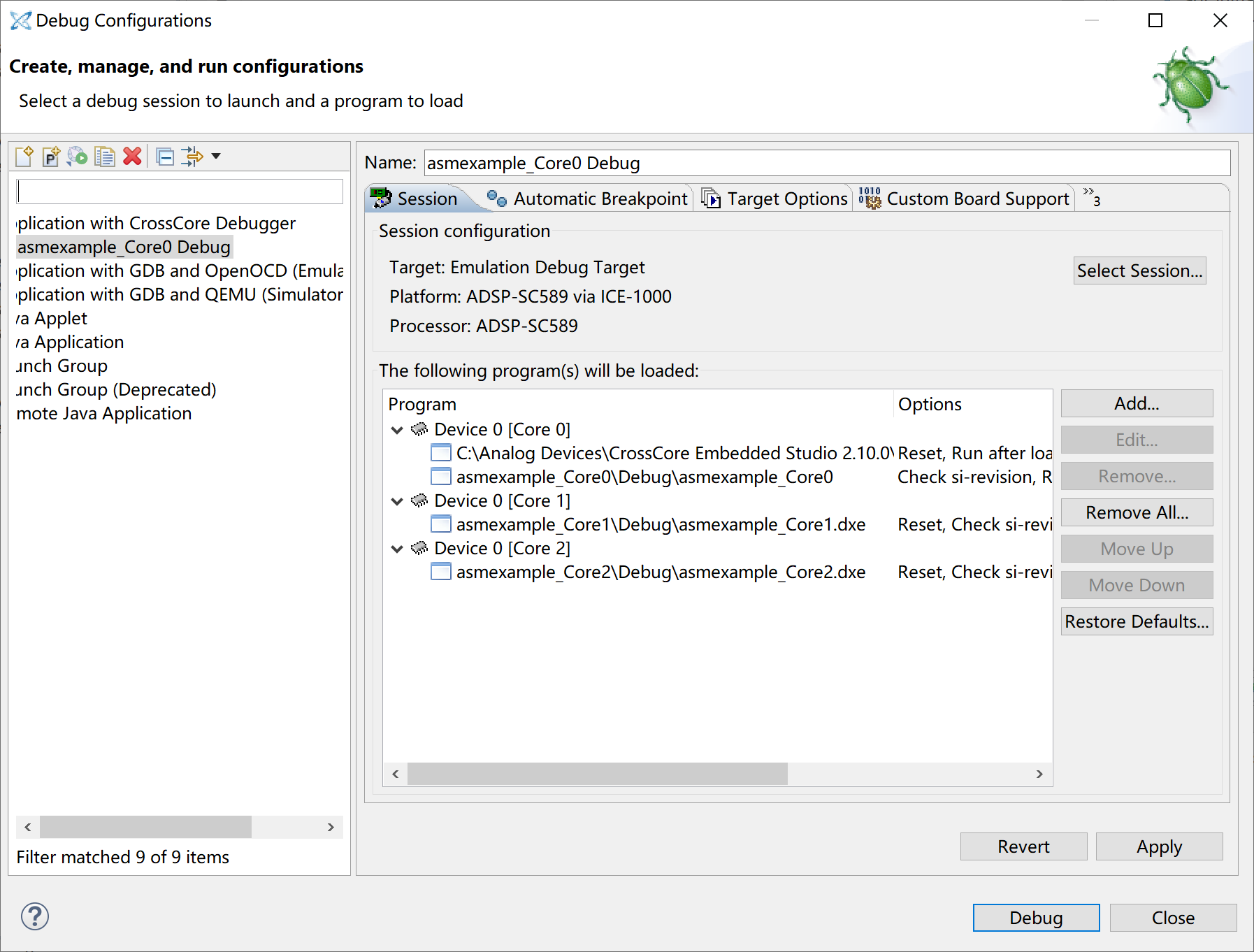Now click the Debug button to launch the debugging session. Once it has started, it should stop at the main function in the ARM core. (Switch to the Debug tab in the left to see which core is currently at.) Click Resume (F5), then 2 DSP cores should be released and start running. Click on Core 1 on the left, it should now also stop at the main function. Click Resume (F5) to let the Core 1 run. It should then print out the message and stop.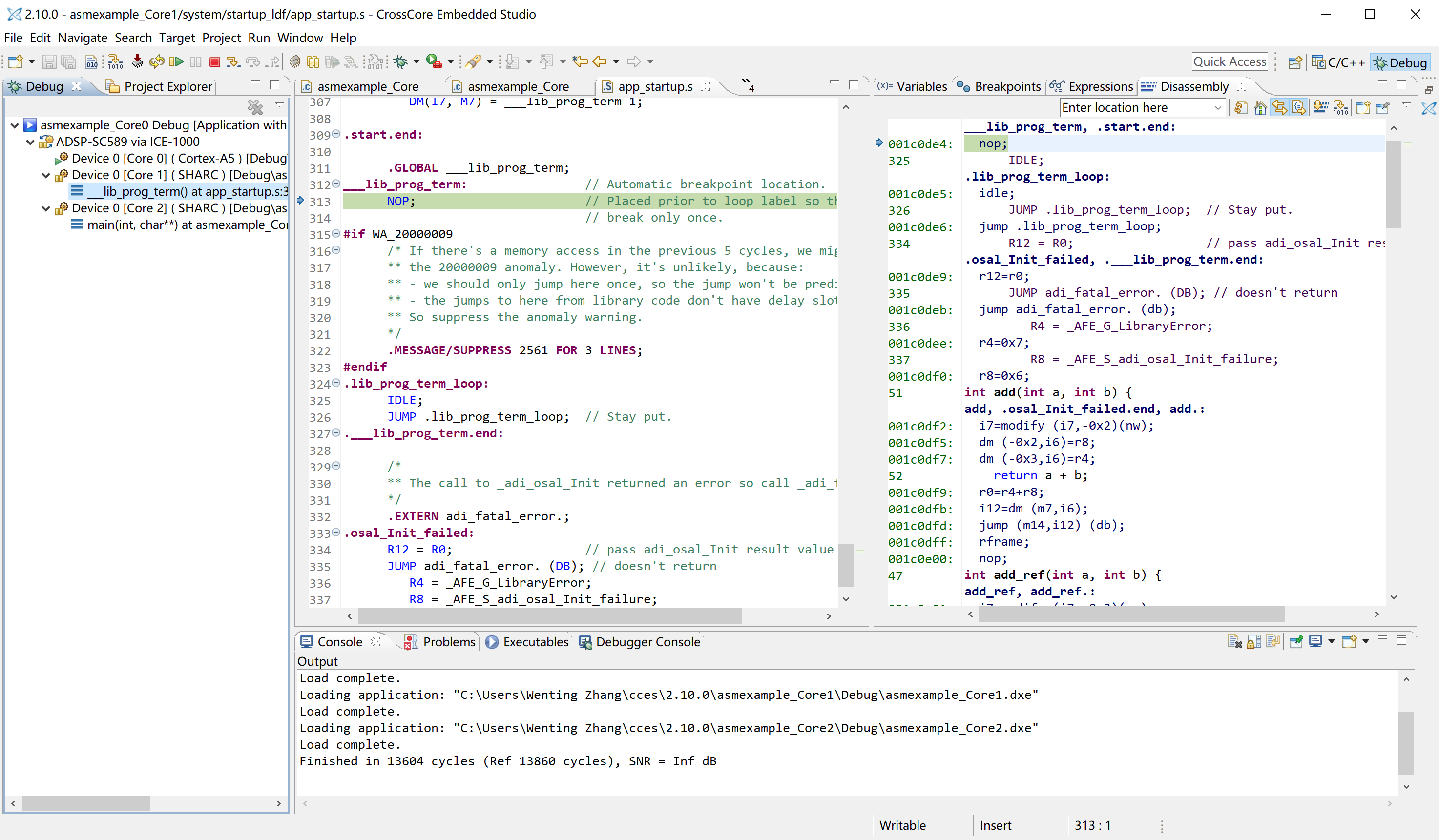We could see the SNR is +Inf dB, which means the output of the two functions is identical. This is expected, as both functions are doing exactly the same thing after all. Once we have the framework up, we could move forward to write some assembly code.

## Assembly Version

The next step would be to replace the C version of the add function with the assembly version. Create a new file called add.ASM in Core1's src folder, and paste in the following code:

``````// add.asm
#include <asm_sprt.h>

.section/pm seg_pmco;
leaf_entry;
// int add(int a, int b);
// Parameters:
// int a : r4
// int b : r8
// Return value:
// int   : r0
r0 = r4 + r8;
leaf_exit;
``````

The code should be fairly easy to understand. As discussed in calling convention, parameters are passed in r4 and r8 registers. The result are passed back in r0 register.

Several notes here. I am using the macro `leaf_entry` and `leaf_exit`at the beginning and end of the function, and this is the recommended way to enter and exit the function when using CCES C runtime. The function name is defined to be "add.", don't forget the dot at the end of the name. This is to comply with the naming convention of the C compiler, so the linker could find the function.

Finally, change the add function definition in add_test.c to a simple extern definition:

```extern int add(int a, int b);
```

Make sure the compilier optimization is enabled in the project properties (disabled by default):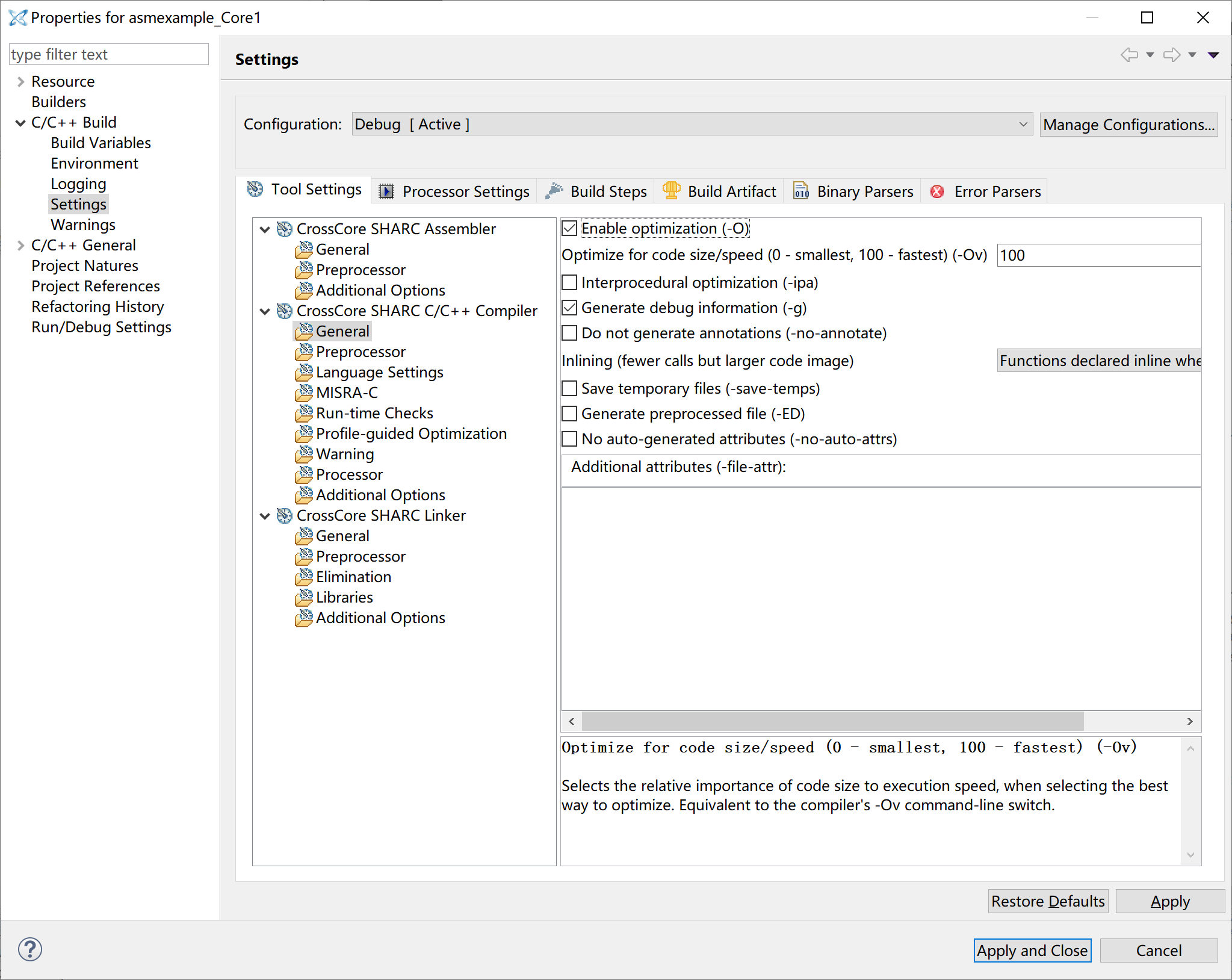Run the program again, and you should see the following output:

``````Finished in 7434 cycles (Ref 5144 cycles), SNR = Inf dB
``````

Great, now it works and passes the test. The performance difference comes from the details hidden by leaf_entry and leaf_exit. But generally, calling a short function like this inside a loop is a bad idea and is avoided anyway. So we don't usually care too much about the function calling overhead. Because I haven't introduced the loop in SHARC assembly yet, so for now we will keep the look outside the function.

# Conclusion

In this part, I introduced the basics of SHARC assembly so you could start writing some code. Next time I will introduce the memory access, conditional execution, and loop. So by that time, you should be able to write more useful stuff with SHARC assembly. Until next time, thanks for reading.